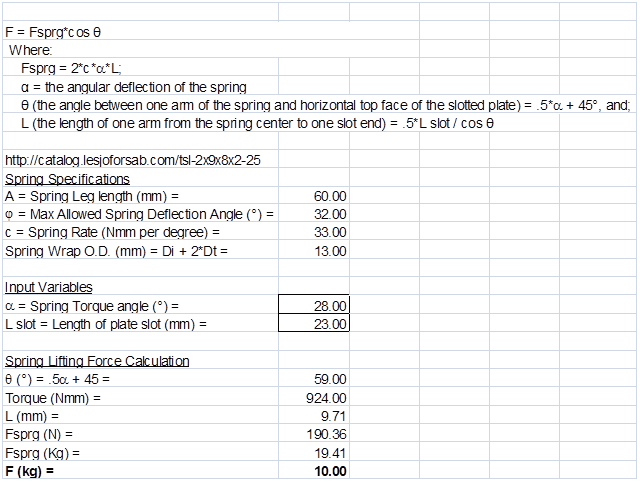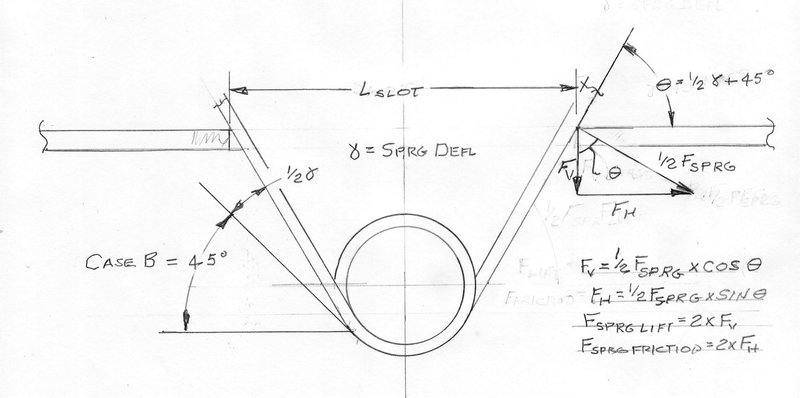# Torsion spring pull force

• mickeysceme

#### mickeysceme

Hi guys,
I am working on design where I am using a torsion spring to hold a certain load.
How can I calculate if the spring I am designing is strong enough to hold the load and prevent it from slipping down?
This is music wire 2mm diam spring, 90deg travel, 60mm legs. Please let me know if I need more information.

#### Attachments

Do you have any spring rate, i.e lb/degree deflection, data for the spring?

Even with that data, if there is going to be any vertical motion on the spring in operation that is going to make it be very difficult to apply that data because as the angle between the plate slot ends changes then the vertical and horizontal load vectors are going to vary throughout the vertical travel of the spring so you will not have a constant spring rate.

Springs of this design are generally used for pivoting components where the increase in spring force can be directly related the their change in angle.

•mickeysceme
Hi @JBA ,

This is the data I have on this spring.
Any idea how can I use?

Thanks

#### Attachments

I would like to do a quick and rough one shot analysis for your load with this spring. How much weight does the spring need to support; and what is the angle between the legs at no load, i.e. 180° or other?

Edit: Do they have an online catalog for these springs; and if so can you give me the web address for that item? The catalog might help answer some of my basic questions about the spring.

Last edited:
I would like to do a quick and rough one shot analysis for your load with this spring. How much weight does the spring need to support; and what is the angle between the legs at no load, i.e. 180° or other?

Edit: Do they have an online catalog for these springs; and if so can you give me the web address for that item? The catalog might help answer some of my basic questions about the spring.
The initial angle between legs is 90 degrees. The weight the spring supports is 10 kg.
Thanks

http://catalog.lesjoforsab.com/tsl-2x9x8x2-25

This is the link to the spring I am talking about. The only thing I did, I added two bends to legs. Cheers

A quick and dirty spreadsheet calculation shows a maximum hanging mass of about 8 kg before extending past the leg length of 60mm. I wouldn't hang my hat on these numbers, but you might be a bit undersized.

#### Attachments

• Sprung Spring.xlsx
43.5 KB · Views: 320
•mickeysceme
At the same time, in starting to analyze the problem I have come to realize that by using a 90° spring configuration you are already placing yourself at a disadvantage because the force vector of a deflected arm is directly perpendicular to the arm and the resulting resisting force is the vertical component of that vector. In equation form the direct spring lifting force for each arm = Fsprg*cos θ, where θ is the angle between the horizontal top of your plate and one arm of the spring. You might be better served by using a style "a" flat spring that would load faster with less lifting force loss due the initial leg angles in your current spring.

In addition to the direct lifting force of the spring there is also a horizontal component of F friction = F*sin θ * "the friction coefficient of the spring material against the plate material (for dry stl on dry stl, is around .57)". The result is that while the direct lifting force decreases with spring deflection, the friction force that also acts to resist the 10k load increases; however, I have to caution you that your design would be best based upon the direct lifting force without depending upon that friction component.

With all of that said, the formula for the spring total lifting force at a deflection angle is F = Fsprg*cos θ
Where: Fsprg = 2*c*α*L;
α = the angular deflection of the spring
θ (the angle between one arm of the spring and horizontal top face of the slotted plate) = α + 45°, and;
L (the length of one arm from the spring center to one slot end) = .5*L slot / cos θ
Using that as a basis I performed the Excel analysis of your current spring with the result shown as a picture below:

Note: I am only including the picture of the analysis rather than uploading the actual excel file because some members of the forum are uncomfortable about posting an actual Excel file on a thread; but, if you feel safe opening this type of file then I will be glad upload the Excel analysis for your review and use.#### Attachments

•mickeysceme
Hi @JBA ,

Is there any possibility that you can add a sketch or diagram how the forces are working on the spring in my case?

At the same time, in starting to analyze the problem I have come to realize that by using a 90° spring configuration you are already placing yourself at a disadvantage because the force vector of a deflected arm is directly perpendicular to the arm and the resulting resisting force is the vertical component of that vector. In equation form the direct spring lifting force for each arm = Fsprg*cos θ, where θ is the angle between the horizontal top of your plate and one arm of the spring. You might be better served by using a style "a" flat spring that would load faster with less lifting force loss due the initial leg angles in your current spring.

In addition to the direct lifting force of the spring there is also a horizontal component of F friction = F*sin θ * "the friction coefficient of the spring material against the plate material (for dry stl on dry stl, is around .57)". The result is that while the direct lifting force decreases with spring deflection, the friction force that also acts to resist the 10k load increases; however, I have to caution you that your design would be best based upon the direct lifting force without depending upon that friction component.

With all of that said, the formula for the spring total lifting force at a deflection angle is F = Fsprg*cos θ
Where: Fsprg = 2*c*α*L;
α = the angular deflection of the spring
θ (the angle between one arm of the spring and horizontal top face of the slotted plate) = α + 45°, and;
L (the length of one arm from the spring center to one slot end) = .5*L slot / cos θ
Using that as a basis I performed the Excel analysis of your current spring with the result shown as a picture below:

Note: I am only including the picture of the analysis rather than uploading the actual excel file because some members of the forum are uncomfortable about posting an actual Excel file on a thread; but, if you feel safe opening this type of file then I will be glad upload the Excel analysis for your review and use.

View attachment 227486

A quick and dirty spreadsheet calculation shows a maximum hanging mass of about 8 kg before extending past the leg length of 60mm. I wouldn't hang my hat on these numbers, but you might be a bit undersized.

Thank a lot for the spreadsheet you have sent.
Can you share the formulas you used for this calculation and diagram of forces?

Attached is a quick force diagram showing how the spring deflection force is divided between a vertical lifting force on your load and a horizontal friction force between the spring and the slot edge. I hope this helps. Unfortunately, an expanded version of my original analysis (that accurately reflects the spring geometry shown this diagram) shows that your current spring selection is not a good fit for your load requirement. Even at the maximum allowed spring deflection, the required length of the legs is so short that less than 1/2 of the spring coil would extend below the surface of your slotted plate.#### Attachments

Attached is a quick force diagram showing how the spring deflection force is divided between a vertical lifting force on your load and a horizontal friction force between the spring and the slot edge. I hope this helps. Unfortunately, an expanded version of my original analysis (that accurately reflects the spring geometry shown this diagram) shows that your current spring selection is not a good fit for your load requirement. Even at the maximum allowed spring deflection, the required length of the legs is so short that less than 1/2 of the spring coil would extend below the surface of your slotted plate.

View attachment 227851
Hi @JBA,
Thank you very much for your help.
I will do some tests with samples once I get them.
Cheers

Just as a note, with your current spring the plate slot length calculates to be no more than 24 mm (15/16") for the lifting force of the spring to equal your 10 Kg load. In any testing you will find that due to arm to slot end friction a longer slot may support your load; but, as I stated in an earlier post, to be safe I would recommend designing the assembly to provide a full 10 Kg direct lifting force ignoring the friction effect.

•mickeysceme
At the same time, in starting to analyze the problem I have come to realize that by using a 90° spring configuration you are already placing yourself at a disadvantage because the force vector of a deflected arm is directly perpendicular to the arm and the resulting resisting force is the vertical component of that vector. In equation form the direct spring lifting force for each arm = Fsprg*cos θ, where θ is the angle between the horizontal top of your plate and one arm of the spring. You might be better served by using a style "a" flat spring that would load faster with less lifting force loss due the initial leg angles in your current spring.

In addition to the direct lifting force of the spring there is also a horizontal component of F friction = F*sin θ * "the friction coefficient of the spring material against the plate material (for dry stl on dry stl, is around .57)". The result is that while the direct lifting force decreases with spring deflection, the friction force that also acts to resist the 10k load increases; however, I have to caution you that your design would be best based upon the direct lifting force without depending upon that friction component.

With all of that said, the formula for the spring total lifting force at a deflection angle is F = Fsprg*cos θ
Where: Fsprg = 2*c*α*L;
α = the angular deflection of the spring
θ (the angle between one arm of the spring and horizontal top face of the slotted plate) = α + 45°, and;
L (the length of one arm from the spring center to one slot end) = .5*L slot / cos θ
Using that as a basis I performed the Excel analysis of your current spring with the result shown as a picture below:

Note: I am only including the picture of the analysis rather than uploading the actual excel file because some members of the forum are uncomfortable about posting an actual Excel file on a thread; but, if you feel safe opening this type of file then I will be glad upload the Excel analysis for your review and use.

View attachment 227486

Do you still have the excel file for this ?